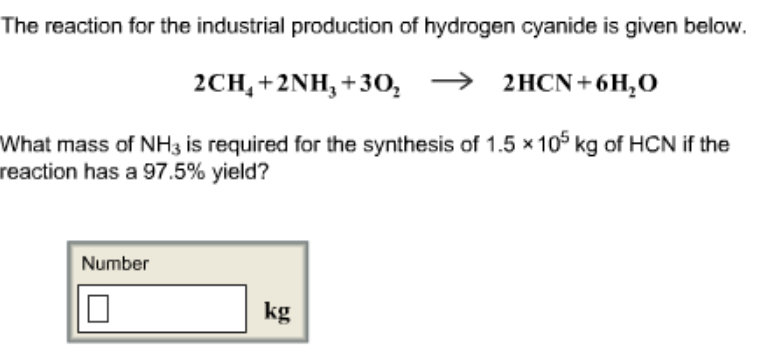# Problem: The reaction for the industrial production of hydrogen cyanide is given below. 2CH4 + 2NH3 + 3O2 --&gt;2HCN + 6H2O What mass of NH3 is required for the synthesis of 1.5 x 105 kg of HCN if the reaction has a 97.5% yield?

###### FREE Expert Solution
97% (287 ratings)###### Problem Details

The reaction for the industrial production of hydrogen cyanide is given below.

2CH4 + 2NH3 + 3O2 -->2HCN + 6H2

What mass of NH3 is required for the synthesis of 1.5 x 105 kg of HCN if the reaction has a 97.5% yield?